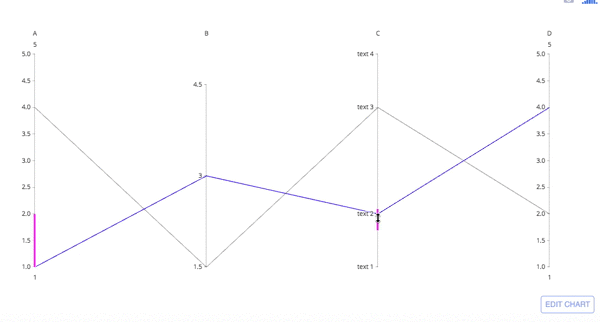Show Sidebar Hide SidebarParallel Coordinates Plot in Python

How to make parallel coorindates plots in Python with Plotly.

Parallel Coordinates plot with plotly express¶

Plotly Express functions take as a first argument a tidy pandas.DataFrame. In a parallel coordinates plot with px.parallel_coordinates, each row of the DataFrame is represented by a polyline mark which traverses a set of parallel axes, one for each of the dimensions. For other representations of multivariate data, also see radar charts and scatterplot matrix (SPLOM).

In :
import plotly.express as px
iris = px.data.iris()
fig = px.parallel_coordinates(iris, color="species_id", labels={"species_id": "Species",
"sepal_width": "Sepal Width", "sepal_length": "Sepal Length",
"petal_width": "Petal Width", "petal_length": "Petal Length", },
color_continuous_scale=px.colors.diverging.Tealrose,
color_continuous_midpoint=2)
fig.show()

Parallel coordinates are richly interactive by default. Drag the lines along the axes to filter regions.

Select the columns to be represented with the dimensions parameter.

In :
import plotly.express as px
iris = px.data.iris()
fig = px.parallel_coordinates(iris, color="species_id",
dimensions=['sepal_width', 'sepal_length', 'petal_width',
'petal_length'],
color_continuous_scale=px.colors.diverging.Tealrose,
color_continuous_midpoint=2)
fig.show()

Parallel Coordinates Chart with go.Parcoords¶

In :
import plotly.graph_objects as go

fig = go.Figure(data=
go.Parcoords(
line_color='blue',
dimensions = list([
dict(range = [1,5],
constraintrange = [1,2], # change this range by dragging the pink line
label = 'A', values = [1,4]),
dict(range = [1.5,5],
tickvals = [1.5,3,4.5],
label = 'B', values = [3,1.5]),
dict(range = [1,5],
tickvals = [1,2,4,5],
label = 'C', values = [2,4],
ticktext = ['text 1', 'text 2', 'text 3', 'text 4']),
dict(range = [1,5],
label = 'D', values = [4,2])
])
)
)
fig.show()

Parallel coordinates are richly interactive by default. Drag the lines along the axes to filter regions and drag the axis names across the plot to rearrange variables.Basic Parallel Coordinates Plot¶

In :
import plotly.graph_objects as go

import pandas as pd

fig = go.Figure(data=
go.Parcoords(
line = dict(color = df['species_id'],
colorscale = [[0,'purple'],[0.5,'lightseagreen'],[1,'gold']]),
dimensions = list([
dict(range = [0,8],
constraintrange = [4,8],
label = 'Sepal Length', values = df['sepal_length']),
dict(range = [0,8],
label = 'Sepal Width', values = df['sepal_width']),
dict(range = [0,8],
label = 'Petal Length', values = df['petal_length']),
dict(range = [0,8],
label = 'Petal Width', values = df['petal_width'])
])
)
)

fig.update_layout(
plot_bgcolor = 'white',
paper_bgcolor = 'white'
)

fig.show()

Advanced Parallel Coordinates Plot¶

In :
import plotly.graph_objects as go

import pandas as pd

fig = go.Figure(data=
go.Parcoords(
line = dict(color = df['colorVal'],
colorscale = 'Electric',
showscale = True,
cmin = -4000,
cmax = -100),
dimensions = list([
dict(range = [32000,227900],
constraintrange = [100000,150000],
label = "Block Height", values = df['blockHeight']),
dict(range = [0,700000],
label = 'Block Width', values = df['blockWidth']),
dict(tickvals = [0,0.5,1,2,3],
ticktext = ['A','AB','B','Y','Z'],
label = 'Cyclinder Material', values = df['cycMaterial']),
dict(range = [-1,4],
tickvals = [0,1,2,3],
label = 'Block Material', values = df['blockMaterial']),
dict(range = [134,3154],
visible = True,
label = 'Total Weight', values = df['totalWeight']),
dict(range = [9,19984],
label = 'Assembly Penalty Wt', values = df['assemblyPW']),
dict(range = [49000,568000],
label = 'Height st Width', values = df['HstW'])])
)
)
fig.show()

Reference¶

See https://plot.ly/python/reference/#parcoords for more information and chart attribute options!